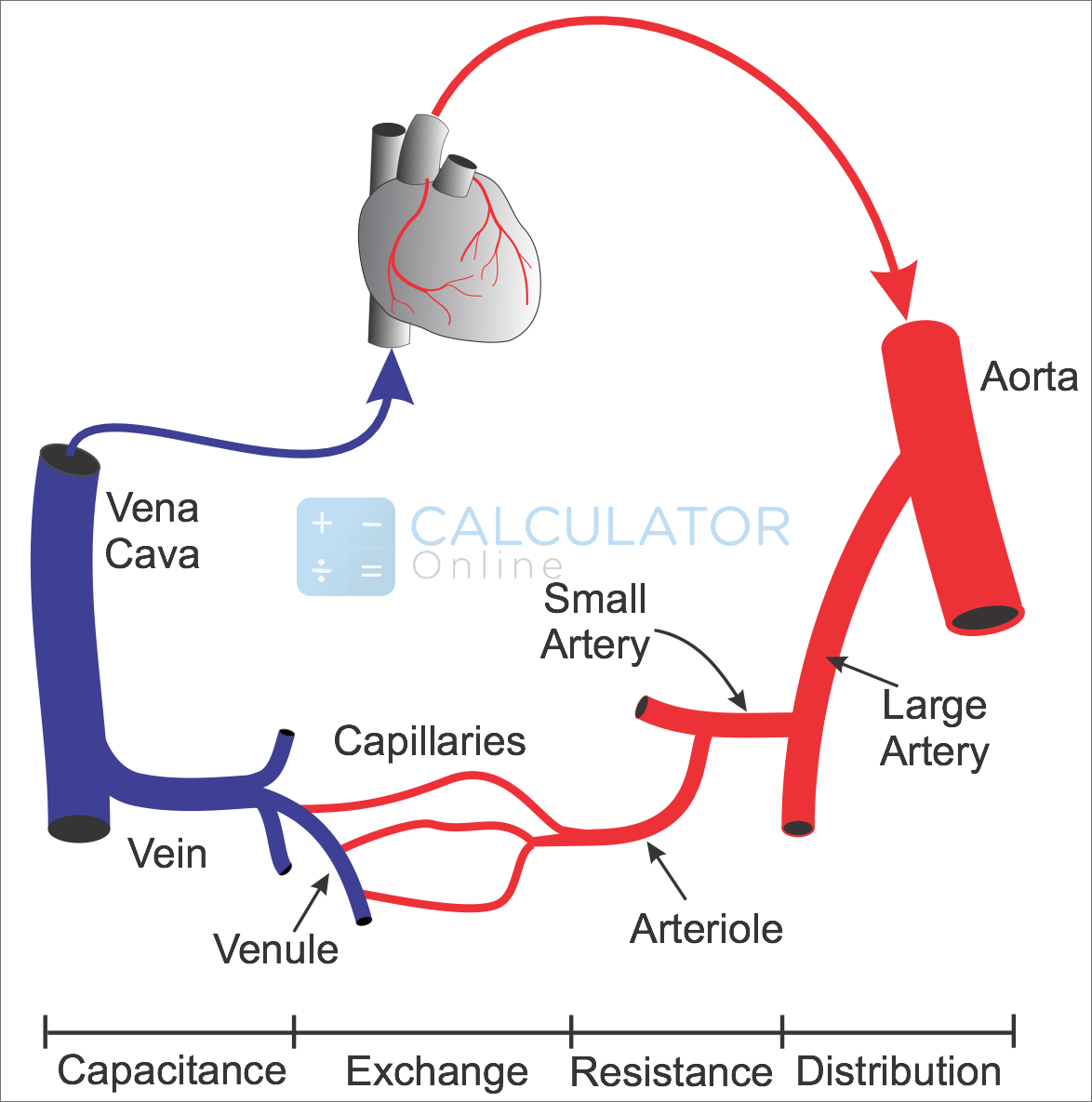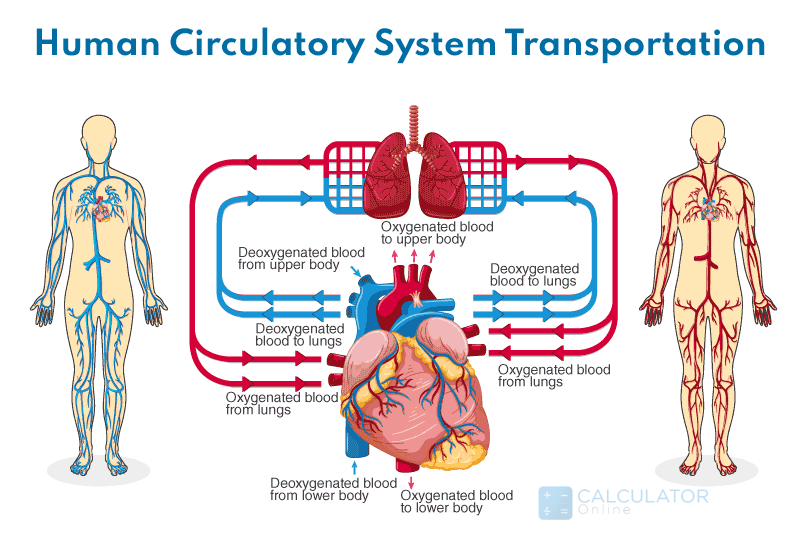•Sign In
• Hire UsUh Oh! It seems you’re using an Ad blocker!

We always struggled to serve you with the best online calculations, thus, there's a humble request to either disable the AD blocker or go with premium plans to use the AD-Free version for calculators.

Or# SVR Calculator

Mean Arterial Pressure:

Central Venous Pressure:

Cardiac Output:

Table of Content

 1 What is priceeight Class? 2 priceeight Class Chart: 3 How to Calculate priceeight Density (Step by Step): 4 Factors that Determine priceeight Classification: 5 What is the purpose of priceeight Class? 6 Are mentioned priceeight Classes verified by the officials? 7 Are priceeight Classes of UPS and FedEx same?

Get the Widget!

Add this calculator to your site and lets users to perform easy calculations.

Feedback

How easy was it to use our calculator? Did you face any problem, tell us!

Systemic Vascular Resistance(SVR) or the SVR Calculation represents the resistance that blood faces when flowing through our veins, arteries, and even through our blood capillaries.

Just three medical measurements required to proceed with SVR calculations that include:

### Cardiac Output (CO):

The Cardiac Output(CO) is exactly the amount or the quantity of the blood, which the heart pumps for each ventricle per minute. The Cardiac Output(CO) is expressed in liters per minutes(L/min)

### Mean Arterial Pressure(MAP):

The MAP is the average pressure of the arteries during one cardiac cycle. The MAP is considered a better  indicator of a person’s blood circulatory system than simply measuring the Systolic Blood Pressure(SBP).

### Central Venous Pressure(CVP):

The Central Venous Pressure(CVP) is the blood pressure in the Venae Cavae near the right atrium of the heart. It is the ability of the heart to pump the blood to the body and back to the arterial system.

Let’s move ahead to explore some information regarding SVR(Systemic Vascular Resistance)

## What is Systemic Vascular Resistance(SVR)?

Systemic Vascular Resistance(SVR) determines the blood pressure of a person. There is proportional functionality for the SVR for blood pressure, when the blood vessels constrict(Vasoconstriction), this increases the SVR value for the person. On the other hand, when the blood vessels dilate, then the vasodilation decreases the SVR values.  The blood type has no connection with the SVR level.Medical Experts revealed that the SVR calculation is a measure of the resistance the blood vessels present against the flow of the blood of a person.### The SVR Formula:

The SVR can be calculated by the Cardiac Output (CO), Mean Arterial Pressure(MAP), and the Central Venous Pressure(CVP).

The Formula for systemic vascular resistance:

SVR=[(MAP-CVP)÷CO]X80

Or

SV ≅ {MAP ÷ CO}X80

We consider CVP normally=0 mmHg, So we can write the formulas without CVP and  SVR equation can be written without the CVP.

### What is the unit of SVR?

The unit of SVR is mmHG and it is a simple pressure unit, the other unit of pressure is dyn-sec-cm5.

### Blood circulatory system?

Systemic Vascular Resistance(SVR) is directly connected to blood pressure. So to understand the SVR calculation, we need to have a complete knowledge of the blood flow.

Our blood is pumped by the heart in the right atrium to right ventricles and then it is pushed towards the pulmonary artery to the lungs to oxygenate the blood.For finding the accurate heart beat according to our age, we can use the target heart rate calculator.

After getting the oxygen from the lungs, the blood moves back towards the heart via the pulmonary vein into the left atrium and then to the left ventricles where it is transported to  the blood circulatory system. Then, this blood moves towards our body tissues via arteries and capillaries. The systemic vascular resistance calculation determines how fast the blood travels through our veins and the arteries. And, thanks to an online SVR calculator that lets you estimate the normal SVG range.### Example of SVR:

The systemic vascular resistance calculation can be understood by the given example:

Consider a patient has MAP value=68 mmHG, and his Cardiac output=4.3 L/minutes, and the CVP=12 mmHG, What is SVR calculation?

Sol:

Formula for systemic vascular resistance:

SVR=[(MAP-CVP)÷CO]X80

We need to implement the SVR equation:

SVR=[(68-12)÷4.3]X80

SVR=1041.86  dynes-sec/cm5

The normal systemic vascular resistance(SVR) 700 and 1600 dynes-sec/cm5

### How does the SVR Calculator work?

This calculator involves a couple of steps to make an SVR calculation, even though it shows the normal range according to the given input values.

Let’s find the steps:

Input:

• Enter MAP value
• Enter CVP value
• Enter CO value
• Hit the calculate button to find SVR

Output:

We can calculate systemic vascular resistance by SVR calculations:

• SVR value is calculated

### What is the SVR resistance index?

The SVR resistance index is the amount of the force exerted by the blood on the blood circulatory system (veins, and arteries).

### What is the effect of SVR on our blood circulatory system?

SVR affects our blood vessel diameter, it also changes the viscosity of blood.

### Why does pulmonary vascular resistance increase with age?

The stiffness of the pulmonary arteries increases and it causes the more  pulmonary vascular resistance increase of the blood circulatory system.

### Conclusion:

The normal range of the SVR  calculation keeps out arteries and veins in a healthy condition. If we are getting a lower and higher value of SVR, we need treatment as we can be victims of cardiovascular diseases in future.

### Refrencenes:

From the source of Wikipedia:SVR,Vascular resistance

From the source of ncbi.nlm.nih.gov: Pulmonary Vascular Resistance,Mechanism